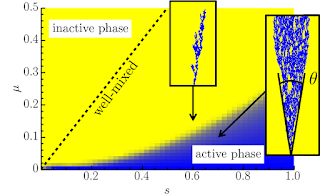## Range expansions

I'm interested in the evolution of populations that are invading or spreading into new territory.  Such populations are called range expansions.A range expansion of E. coli on a Petri dish. (Image courtesy of Bryan Weinstein)
When populations spread on a surface or in three-dimensional space (such as a solid tumor in healthy tissue), spatial fluctuations may play an important role in the evolutionary dynamics.  We can see in the image above that an initially well-mixed population of red, blue, and green-fluorescent E. coli segregate into single-colored sectors as the organisms spread across the surface of a Petri dish (forming a colony).This is a result of the small effective population size at the frontier of the colony, where a small fraction of the total population divides into the new territory.  Small number fluctuations, then, rapidly fix the population to a single color at the frontier.

We studied such range expansions in the presence of selection and mutation.  In the simplest instance, we consider a blue strain with a selective advantage $$s$$ over a yellow strain, which may convert to the yellow strain with some rate  $$\mu$$.  In this case, depending on the mutation rate $\mu$ and the selection coefficient $$s$$, the blue strain may either survive in the population at long times, or go extinct.  Spatial fluctuations strongly suppress the blue strain, leading to more extinction, as shown in the phase diagram below.In a two-dimensional range expansion (a microbial colony growing across a Petri dish, for example), extinction is enhanced due to spatial fluctuations.  We see in this phase diagram that a blue strain with a selective advantage $$s$$ will go extinct faster in a range expansion for a fixed mutation rate $\mu$ than in the well-mixed case (dashed line).  Such spatial extinction transitions may be described by directed percolation, which has concrete predictions for quantities such as the opening angle $\theta$ of blue genetic sectors [1,2].

## Domain walls in ferroelectrics and cellular populations

Remarkably, domain walls in ferroelectric materials (regions separating two different electric polarizations) share similar characteristics as range expansion frontiers or interfaces between competing species. In both cases, the interface can be captured by a scalar function $$\phi(\mathbf{x},t)$$ that indicates the "phase" of the material, such as the polarization $$P$$ or the cellular density. Then, the dynamics of this field are governed by an equation that tends to minimize some effective "free energy" $$\mathcal{F}$$, which has the general form
$$\mathcal{F}=\int \mathrm{d}\mathbf{x}\left[ \kappa (\nabla \phi)^2 + V(\phi) \right],$$
where $V(\phi)$ has local minima at the preferred values of $\phi$, which might be the two stable polarizations $\pm P_0$ along some axis for a uniaxial ferroelectric material, or simply minima at $$\phi=0,1$$ for the two stable states of a growing population (no cells and cells at the local carrying capacity for which we can take $$\phi=1$$ without loss of generality). The simplest evolution for $$\phi(\mathbf{x},t)$$ would be the "model A"  dynamics
$$\partial_t \phi = - \nu\, \frac{\delta \mathcal{F}}{\delta \phi} + \xi(\mathbf{x},t),$$
where $\nu$ is a "viscosity" setting the timescale of relaxation toward equilibrium and $\xi(\mathbf{x},t)$ is a spatiotemporal noise representing either thermal fluctuations for ferroelectric materials, or stochastic cell birth/death for cellular populations. These different sources of noise have significant consequences on the noisy dynamics of the wall. The characteristic features of the noise are specified by the noise correlations, which are given by $$\langle \xi(\mathbf{x},t) \xi(\mathbf{x}',t') \rangle = \Xi[ \phi ] \delta(\mathbf{x}-\mathbf{x}') \delta(t-t')$$, where $\Xi$ is a constant for thermal noise (proportional to the temperature and $\nu$ according to the fluctuation-dissipation theorem) and proportional to $\phi$ in the case of cellular populations as no (local) stochastic birth/death can happen in the absence of cells.

The dynamics may also be significantly influenced by quenched noise, such as obstacles or nutrient variation for cellular growth or crystalline defects in ferroelectric materials. Such noise is captured by introducing random variations in the coefficients of the potential $$V[\phi]$$. The thermal and quenched noises have very different effects, with quenched noise tending to pin domain walls and thermal noise tending to broaden and depin the domain wall. These effects, however, also depend on the nature of the potential $$V[\phi]$$. In ferroelectrics, for example, there may exist other metastable states (such as a metastable paraelectric state with $$P=0$$). These states may be excited by the thermal fluctuations, leading to dramatic changes to the domain wall behavior .

References
 M. O. Lavrentovich, M. E. Wahl, D. R. Nelson, and A. W. Murray Spatially constrained growth enhances conversional meltdown Biophysical Journal 110(12)  271 (2016)
 M. O. Lavrentovich, K. S. Korolev, and D. R. Nelson Radial Domany-Kinzel models with mutation and selection Physical Review E 87 012103 (2013)
 P. C. Hohenberg and B. I. Halperin Theory of Dynamics Critical Phenomena Reviews of Modern Physics 49, 435 (1977)
 N. Bauer, S. M. Neumayer, P. Maksymovych, M. O. Lavrentovich (arxiv.org/abs/2208.02990) (2022)